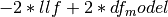# statsmodels.tsa.arima_model.ARMAResults¶

class `statsmodels.tsa.arima_model.``ARMAResults`(model, params, normalized_cov_params=None, scale=1.0)[source]

Class to hold results from fitting an ARMA model.

Parameters: model : ARMA instance The fitted model instance params : array Fitted parameters normalized_cov_params : array, optional The normalized variance covariance matrix scale : float, optional Optional argument to scale the variance covariance matrix. Attributes aic : float Akaike Information Criterionwhere df_model includes all AR parameters, MA parameters, constant terms parameters on constant terms and the variance. arparams : array The parameters associated with the AR coefficients in the model. arroots : array The roots of the AR coefficients are the solution to (1 - arparams*z - arparams*z**2 -...- arparams[p-1]*z**k_ar) = 0 Stability requires that the roots in modulus lie outside the unit circle. bic : float Bayes Information Criterion -2*llf + log(nobs)*df_model Where if the model is fit using conditional sum of squares, the number of observations nobs does not include the p pre-sample observations. bse : array The standard errors of the parameters. These are computed using the numerical Hessian. df_model : array The model degrees of freedom = k_exog + k_trend + k_ar + k_ma df_resid : array The residual degrees of freedom = nobs - df_model fittedvalues : array The predicted values of the model. hqic : float Hannan-Quinn Information Criterion -2*llf + 2*(df_model)*log(log(nobs)) Like bic if the model is fit using conditional sum of squares then the k_ar pre-sample observations are not counted in nobs. k_ar : int The number of AR coefficients in the model. k_exog : int The number of exogenous variables included in the model. Does not include the constant. k_ma : int The number of MA coefficients. k_trend : int This is 0 for no constant or 1 if a constant is included. llf : float The value of the log-likelihood function evaluated at params. maparams : array The value of the moving average coefficients. maroots : array The roots of the MA coefficients are the solution to (1 + maparams*z + maparams*z**2 + ... + maparams[q-1]*z**q) = 0 Stability requires that the roots in modules lie outside the unit circle. model : ARMA instance A reference to the model that was fit. nobs : float The number of observations used to fit the model. If the model is fit using exact maximum likelihood this is equal to the total number of observations, n_totobs. If the model is fit using conditional maximum likelihood this is equal to n_totobs - k_ar. n_totobs : float The total number of observations for endog. This includes all observations, even pre-sample values if the model is fit using css. params : array The parameters of the model. The order of variables is the trend coefficients and the k_exog exognous coefficients, then the k_ar AR coefficients, and finally the k_ma MA coefficients. pvalues : array The p-values associated with the t-values of the coefficients. Note that the coefficients are assumed to have a Student’s T distribution. resid : array The model residuals. If the model is fit using ‘mle’ then the residuals are created via the Kalman Filter. If the model is fit using ‘css’ then the residuals are obtained via scipy.signal.lfilter adjusted such that the first k_ma residuals are zero. These zero residuals are not returned. scale : float This is currently set to 1.0 and not used by the model or its results. sigma2 : float The variance of the residuals. If the model is fit by ‘css’, sigma2 = ssr/nobs, where ssr is the sum of squared residuals. If the model is fit by ‘mle’, then sigma2 = 1/nobs * sum(v**2 / F) where v is the one-step forecast error and F is the forecast error variance. See nobs for the difference in definitions depending on the fit.

Methods

 `aic`() `arfreq`() Returns the frequency of the AR roots. `arparams`() `arroots`() `bic`() `bse`() `cov_params`() `fittedvalues`() `forecast`([steps, exog, alpha]) Out-of-sample forecasts `hqic`() `llf`() `mafreq`() Returns the frequency of the MA roots. `maparams`() `maroots`() `plot_predict`([start, end, exog, dynamic, ...]) Plot forecasts `predict`([start, end, exog, dynamic]) ARMA model in-sample and out-of-sample prediction `pvalues`() `resid`() `summary`([alpha]) Summarize the Model `summary2`([title, alpha, float_format]) Experimental summary function for ARIMA Results

Attributes

 `use_t`